## CONLANG@LISTSERV.BROWN.EDU

#### View:

 Message:[First|Previous|Next|Last] By Topic:[First|Previous|Next|Last] By Author:[First|Previous|Next|Last] Font: Proportional Font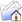LISTSERV Archives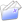CONLANG HomeCONLANG October 2004, Week 3

Subject:Re: Calénnawn and a manic digression on numbers

From:Date:Wed, 20 Oct 2004 11:51:58 -0400

Content-Type:text/plain

Parts/Attachments: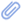text/plain (403 lines)
 ```WOW!!! Gee, thanks, Teoh... very clearly written, and it makes good sense to translate from base nine WITH zero to the novenus without zero. I really appreciate the work you did on that for me. I still find the multiplication the most difficult, and I still can't figure out how adding 33 nine times came up with a number higher than its original in base ten. I thought I had addition down pat. Wish I were more intuitive when it comes to math. I'd like to get hold of David Peterson's zero-less base eight. I wonder if making an even number the base makes a subtle difference. What I discovered about my novenus (a number not chosen arbitrarily...nine is particularly potent) is its groupings of threes, of course; multiples of threes produces perfect numeric symmetry, visually and conceptually; would such a society make a distinction between "even" and "odd" numbers"? Or would triads be the unit? What different mathematical concepts would they come up with? Or could they? Is mathematics pure logic in every basal configuration? Even without zero? Let's try a zero-less "sextus" (the Teonim, after all, have six fingers on one hand): 1 = 2 3 4 5 6 11 = 7 12 = 8 13 = 9 14 = 10 15 = 11 16 = 12 21 = 13 22 = 14 23 = 15 24 = 16 25 = 17 26 = 18 31 = 19 32 = 20 I notice that unlike my novenus, the numbers ending with even digits always express even numbers. Let's try multiples of three: 3, 6, 13, 16, 23, 26, 33, etc. Bravo. Always three or six as the final digit. What would be useful, were I to pursue this, would be a calculator that could do all this for me. But that would take time to construct. Zero is so logical. It evens things out. To go from 19 to 21 seems a little cock-eyed. I wonder if this is how the Arabs discovered it, by fiddling around with a zeroless system first. Sally ----- Original Message ----- From: "H. S. Teoh" <[log in to unmask]> To: <[log in to unmask]> Sent: Monday, October 18, 2004 2:16 PM Subject: Re: Calénnawn and a manic digression on numbers > On Mon, Oct 18, 2004 at 03:26:41AM -0400, Sally Caves wrote: > [...] >> >> In my salad days I experimented with a non-zero enumeration founded on a >> "sort of" base nine, call it a novenus or whatever, but it became >> massively >> unusable, partly because of my poor math skills. That "zero" is >> essential, >> and a great gift from the Arabs, and I can't think numerically without >> it. >> Here were my early attempts: > [...] > > It looks like a pretty good start, to me. > >> I couldn't pursue it. Can't still. The Teonim adopted the zero. Math >> experts out there of COURSE? Who knows what symmetries I may have >> discovered >> had I persisted? What contributions to the mythology of number I may >> have >> created for an alien people fixated on three? I'm SURE somebody else has >> tried this; but my knowledge of math problems is meager, and this system >> seems flawed. > > It doesn't look flawed to me. Keep in mind that you're really counting > in base 9, and it may help to count base 9 using a zero-based system > (with which we are probably more familiar with than a zero-less > system), and then translate this into your zero-less notation. You're > probably having difficulties because you're trying to map base 10 onto > base 9. > > Let's begin by counting in a base 9 system with a zero. In > mathematical lingo, that's "nonal", base 9 (as opposed to "decimal", > base 10). First of all, just as in decimal, base 10, we count from 0 > to 9 rather than 1 to 10 (since we have no single symbol for 10), in > nonal the digits we have are 0 through 8 (there is no "9" in nonal, > nine is written as "10"). Here then, is how one counts in nonal: > > decimal nonal > 0 0 > 1 1 > 2 2 > ... > 8 8 > 9 10 <-- note this divergence > 10 11 > 11 12 > ... > 17 18 > 18 20 <-- note this > 19 21 > ... > > Let's compare this system with decimal and see if we can discern its > underlying workings. In decimal, when we write 27, we mean 2*10 + 7, > since the 2 is in the tens' place, and 7 is in the ones' place. When > we write 123, we mean 1*100 + 2*10 + 3. This is because the 1 is in > the hundreds' place. Notice the sequence: ones, tens, hundreds. Ten is > simply 10 times 1, and a hundred is 10 times 10. A thousand, which is > represented by the 4th decimal place, is 10 times 100. Therefore, we > see that each position to the left represents the next power of 10. So > if we write 12345, that's 5 + 4*10 + 3*100 + 2*1000 + 1*10000. > > In a similar way, in nonal we multiply not by 10, but by 9: when we > write _21_ in nonal, we mean 1 + 2*9 = 19. Similarly, _1234_ in nonal > is 4 + 3*9 + 2*18 + 1*81 = 922 (decimal). > > Let's adopt a notation to keep track of which base we're counting in: > when I write 1234_10, it means "1234" in base 10, which is one > thousand, two hundred and thirty-four. When I write 1234_9, it means > "1234" in base 9, which is nine hundred twenty-two. > > Now, let's do some arithmetic in base 9: > 127_9 > + 32_9 > ------- > ? > > This is done in an analogous way to base 10. We first add 2 to 7, > which is 9. However, in base 9, there is no such digit "9"; hence, we > must carry the 9 to the next place. This is analogous to how in base > 10, if adding two digits gives a number >= 10, we have to carry it to > the next place. So 2 + 7 = 10_9. So in the next place, we have 1 (the > carry) + 2 + 3 = 6. There is no carry from this place. The last place > is easy: 1 + 0 = 1. Hence, the result is: > > 127_9 + 32_9 = 160_9. > > Subtraction works in a similar way. Let's say we have: > 362_9 > - 64_9 > ------- > ? > > We proceed in an analogous way as in decimal: subtract 4 from 2. Well, > 2 is smaller than 4, so we need to borrow. Now, in decimal, we'd > borrow a 10 from the next place; but since this is nonal, we don't > borrow 10, but 9. So we have (9+2) - 4 = 7. This is the first digit of > the answer. In the next place, the 6 has become 5 because we borrowed > a 9 from it. So we need to subtract 6 from 5. But 5 is smaller than 6, > so we again need to borrow. Again, we borrow 9 rather than 10, since > we're counting in nonal. So we have (9+5) - 6 = 8. Finally, in the > last place, we have (3-1) - 0 (because we borrowed a 9 from the 3). So > the final answer is 287_9. > > Multiplication proceeds in the same way. Say we have: > 68 > * 14 > ---- > ? > > First, we multiply 8 by 4, and get 32. Now, in base 10, we'd carry the > 3 and keep the 2. But what we're *really* doing is, we're *dividing* > 32 by 10, and carrying the quotient, keeping the remainder in the > first place. So in base 9, we divide 32 by 9, giving a quotient of 3 > and a remainder of 5. Therefore, we carry 3 and write 5 in the first > position: > 68 > * 14 > ---- > 5 > > Next, we multiply 6 by 1, and add the carry, which is 3. This gives 9. > But since we're counting in nonal, we can't write _9_, so we have to > carry it. This is done by dividing 9 by 9, giving a quotient of 1 and > a remainder of 0. Therefore, the first row in the multiplication is: > 68 > * 14 > ---- > 105 > > Proceeding to the second row, we now multiply 1 by 8, giving 8: > 68 > * 14 > ---- > 105 > 8 > > Continuing, we multiply 6 by 1, giving 6: > 68 > * 14 > ---- > 105 > 68 > > Finally, we add them up: > 68 > * 14 > ---- > 105 > +68 > ---- > 785 > > Therefore, 68_9 * 14_9 = 785_9. > > I'd proceed with long division, but I think you get the idea already. > What we're *really* interested in is, how do we translate nonal into a > zero-less system? This is not too hard once you're familiar with > arithmetic in nonal. > > First of all, there will be no 0, obviously. But if we're gonna count > in base 9, we must have 9 distinct symbols for each digit. This means > we add the digit "9" to the system. Let's adopt the convention that > when I write 123_N, it means the number "123" in novenus. Let's see > how to translate nonal to novenus: > 1_9 -> 1_N > 2_9 -> 2_N > ... > 8_9 -> 8_N > > Here's where it gets interesting. The next number in nonal is 10_9 > (which is nine). In novenus, however, we have no zero, so we can't > write 10_N. But we do have a "9", so we write 9_N instead: > 10_9 -> 9_N > > Keep in mind that 9_N is already in the 2nd place in nonal. So the > next numbers would be: > 11_9 -> 11_N > 12_9 -> 12_N > ... > 18_9 -> 18_N > 20_9 -> 19_N <-- note this transition > 21_9 -> 21_N > 22_9 -> 22_N > ... > > Notice how, except for the powers of 9, the representation of nonal is > identical to the representation in novenus. As long as there are no > 0's in the nonal representation of a number, the novenus number is > exactly the same. Now, what if there *are* zeroes in the nonal? For > example: > 103_9 -> ?_N > > Now, note that 103_9 = 100_9 + 3_9. 100_9 is simply 9*9, which is > eighty-one (81_10). In novenus, this is simply 90_N. So 103_9 = 90_N + > 3_N (because 3_9 = 3_N). This gives us: > > 90_N > + 3_N > ------ > > Let's see how addition is done in novenus. Adding 3 to 0 gives 3: > 90_N > + 3_N > ------ > 3_N > > Next, adding 9 to 0 gives 9: > 90_N > + 3_N > ------ > 93_N > > Therefore, 103_9 = 93_N. Notice that we've essentially just collapsed > the "10" in the nonal to "9" in the novenus. > > Let's see if we can convert novenus back to nonal: > 99_N = ?_9 > > To convert it, we simply note that 9_N = 10_9. So the rightmost "9" is > represented by 0 in the nonal, carry 1. Adding this 1 to the leftmost > "9" gives ten. But ten is larger than 9, so we need to carry it as > well. We do this by dividing by 9, giving us a quotient of 1 and a > remainder of 1. Therefore: > 99_N = 110_9 > > Note that the next number in the sequence is: > 111_N = 111_9. > > (Because adding 1 to 99_N causes the first "9" to become ten, which > requires a carry. Dividing by 9 gives a quotient of 1 and a remainder > of 1. The quotient is carried to the next digit where the same thing > occurs: 9+1 = ten, which needs to be carried; carrying turns it into a > quotient of 1 and a remainder of 1. Hence, 99_N + 1 = 111_N.) > > Let's do one last example: novenus subtraction. > 3121_N > - 1129_N > -------- > ? > > First, we subtract 9 from 1. Since 1 is too small, we borrow a 9 from > the next place. (This is exactly the same as in nonal.) So we have > (9+1) - 9 = 1: > 3121_N > - 1129_N > -------- > 1_N > > Now, in the next place, the 2 has become a 1 because of the borrow. > Now we need to subtract 2 from 1. But 1 is less than 2, so we borrow 9 > from the next digit. This gives us (9+1)-2 = 8. > 3121_N > - 1129_N > -------- > 81_N > > Now, in the third place, the 1 has become a zero because of the > borrow. But we can't have zeroes in novenus, so we must borrow a 9 > from the next place to avoid having to write a zero. We then subtract > 9 from this 9... but we still get a zero. Since we can't write zero in > novenus, we must borrow a *second* time to get another 9: > 3121_N > - 1929_N > -------- > 981_N > > So in the final place, 3 has become 1 because we've borrowed twice > from it. Subtracting 1 makes it vanish; so: > 3121_N > - 1929_N > -------- > 981_N > > There is a tricky special case which I should mention, and that is > when a second borrow would make the last digit smaller than what is > being subtracted from it. For example: > 211_N > - 199_N > ------- > ? > > We proceed as usual: we can't subtract 9 from 1, so we borrow a 9 from > the next place. This gives us (9+1)-9 = 1: > 211_N > - 199_N > ------- > 1_N > > In the second place, the 1 has vanished, because of the borrow; but we > can't write zero in novenus so we must borrow from the 2. This gives > us 9 - 9. But that is still zero, so we borrow again from 2, so the > second digit is now 9: > 211_N > - 199_N > ------- > 91_N > > But now we have a problem. Having borrowed twice from 2, it is now > zero. We can't subtract 1 from 0, and there's nothing left to borrow > from. What do we do now? > > One way to resolve this is to notice that if we *hadn't* borrowed the > second time, we would have ended up with zero in the 2nd place, and 1 > in the third place. Subtracting 1 from 1 makes it zero, so BOTH digits > vanish. Hence, the correct answer is actually: > 211_N > - 199_N > ------- > 1_N > > because both the 2nd and 3rd digits have vanished. And indeed, if we > check using novenus addition, we see that 199_N + 1 = 211_N. > > The same thing may happen with larger numbers, for example: > 21113_N > - 19921_N > --------- > 82_N > > The rightmost 3 digits all vanish. > > >> Are there any natural numeric systems that do this kind of thing? > [...] > > I don't know if there are any *natural* systems that do this, but I > seem to recall some time ago on the list where someone (was it David > Peterson?) devised a zero-less base 8 system along exactly the same > lines. Addition/subtraction in that system works exactly in an > analogous way with what I've described above. > > > T > > -- > Real Programmers use "cat > a.out". > ```

#### Search Archives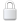Log In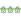Get Password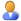Subscribe or Unsubscribe